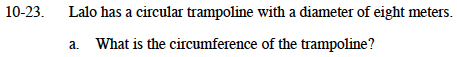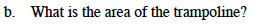### Home > MC1 > Chapter 10 > Lesson 10.1.2 > Problem10-23

10-23.Circumference is equal to 2πr, or the diameter times pi.

C = 8π, or approximately 25.13 meters.Remember that area is equal to πr2. What is the radius of this circular trampoline if the diameter is 8 meters?
(Remember that the radius is equal to half of the diameter.)